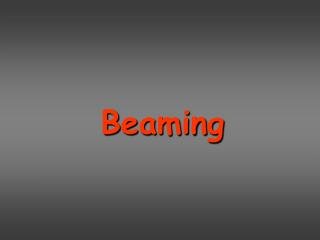DownloadDownload PresentationBeaming

# Beaming

Télécharger la présentation## Beaming

- - - - - - - - - - - - - - - - - - - - - - - - - - - E N D - - - - - - - - - - - - - - - - - - - - - - - - - - -
##### Presentation Transcript

1. Beaming

2. Beaming LHC  ~7 TeV protons  g = 7000

3. TeV blazars 1 TeV

4. Cosmic rays 1020 eV = 108 TeV = 1011 mpc2 = tennis ball at 100 km/s

5. A few milligrams per decade?

6. Radio-loud AGNs Gamma Ray Bursts ~ 10-5 Mo in a few sec G~300 ~ 0.1 Mo yr-1 G~20

7. Text book special relativity Lorentz transformations: v along x x’ = G (x – vt) y’ = y z’ = z t’ = G (t – v x/c2) x = G (x’ + vt’) y = y’ z = z’ t = G (t’ + v x’/c2) for Dt = 0Dx = Dx’/GContraction for Dx’ = 0Dt = G Dt’time dilation To remember: mesons created at a height of ~15 km can reach the earth, even if their lifetime is a few microsec  ct’life=hundreds of meters.

8. Can we see contracted spheres? v=0 G=1 v v=0.866c G=2 Einstein: Yes!

9. v=0 G=1 James Terrel 1959 NO! Rotation, not contraction! Roger Penrose 1959 v v=0.866c G=2

10. Relativity with photons From rulers and clocks to photographs and frequencies Or: from elementary particles to extended objects

11. The moving square b=0 b=0.5 Your camera, very far away

12. The moving square ltot = l’ (b+1/G) max:21/2l’ (diag) min: l’ (for b=0) t=l’/c l’/G vt=bl’

13. l’cosa= bl’  cosa = b cos(p-p/2-a) = sina = 1/G l’ p/2-a

14. a ) p/2-a

15. p/2-a

16. Time Dte = emission time in lab frame Dte’ = emission time in comov. frame Dte = Dte’ G CD = cDte – cDtebcosqDtA= Dte (1-bcosq)  DtA= Dte’ G(1-bcosq)

17. 1 d = G(1-bcosq) Relativistic Doppler factor d DtA= Dte’ G(1-bcosq) n= n’ / G(1-bcosq) Standard relativity You remain in lab frame You change frame Doppler effect

18. 1 d= G(1-bcosq) Relativistic Doppler factor d { 2G for q=0oG for q=1/G 1/Gforq=90o = At small angles, Doppler wins over Spec. Relat.

19. 25 light years in 3 years… the velocity is 8.3 c

20. v=0.99c Nucleo

21. Core

22. Dsapp b sinq vDtesinq bapp = =vapp = DtA 1-bcosq Dte (1-bcosq) There is no G. Correct? q=0o bapp=0 cosq=b; sinq=1/G  bapp=bG q=90obapp=b

23. bapp ~ 30

24. Aberration of light

25. Gravity bends space

26. Aberration of light sinq = sinq’/d dW =dW’/d2

27. Aberration of light v K K’ sinq = sinq’/d dW = dW’/d2

28. I(n) I’(n’) = invariant = n3 n’3 erg E I(n) = = cm2 s Hz sterad dAdt dn dW Observed vs intrinsic Intensity I(n) = d3I’(n’)

29. I(n) I’(n’) = invariant = n3 n’3 erg E I(n) = = cm2 s Hz sterad dAdt dn dW Observed vs intrinsic Intensity I(n) = d3I’(n’)

30. I(n) I’(n’) = invariant = n3 n’3 erg E’d = I(n) = d3I’(n’) = cm2 s Hz sterad dA’dW’/d2 = = I F d4I’ d4F’ Observed vs intrinsic Intensity I(n) = d3I’(n’)

31. v=0 L=100 W

32. v=0.995c G=10 L=0.6mW L=16MW L=10mW

33. v=0.995c G=10 …….? blazars radiogalaxies

34. v=0.995c G=10 blazars! blazars radiogalaxies

35. v counterjet (invisible) jet v

36. A question • Some blob is moving atG>>1, above a black hole of mass M. It is optically thin. • It moves within a region full of radiation produced by the accretion disk. • What is the Eddington luminosity? G

39. Line emission and radiative transitions in atoms and molecules • Breemstrahlung/Blackbody • Curvature radiation • Cherenkov • Annihilation • Unruh radiation • Hawking radiation • Synchrotron • Inverse Compton Radiation processes

40. E V=0

41. E V(g=2) Contracted sphere… E-field lines at time 9.00 point to… where the charge is at 9.00 Charge at time 9.00 Breaking news: what happens with the gravitational field?

42. dP = e2a2sin2Q dW 4p c3 Stop at 8:00 V http://www.cco.caltech.edu/~phys1/java/phys1/MovingCharge/MovingCharge.html

43. Synchrotron

44. d e (gmv) FL = = v x B c dt Synchrotron • Ingredients: Magnetic field and relativistic charges • Responsible: Lorentz force • Curiously, the Lorentz force doesn’t work. q

45. Total losses Pe = P’e P=E/t and E and t Lorentz transform in the same way Please, Pe is not Preceived!!

46. 2e2 2e2 Pe = P’e = a’2 = (a’2 + a’2 ) 3c3 3c3 a’ = g3a a’ = g2a 2e2 Pe = P’e = g4 (g2a2 + a2 ) 3c3 Big? NO! a is small Total losses Why?

47. a|| = 0 a = e v B sinq g mc 2e4 PS(q) = B2g2 b2 sin2q d e (gmv) FL = = v x B 3m2c3 r0=e2/mec2 sT = 8pr0/3 c dt PS(q) = 2sTcUBg2 b2 sin2q 2 <PS> = 4 sTcUBg2 b2 3 g~constant, at least for one gyroradius q=pitch angle If pitch angles are isotropic

48. e v B sinq g mc 2e4 PS(q) = B2g2 b2sin2q d e (gmv) FL = = v x B 3m2c3 r0=e2/mec2 sT = 8pr0/3 c dt 2 <PS> = 4 sTcUBg2 b2 3 a|| = 0 a = PS(q) = 2sTcUBg2 b2sin2q If pitch angles are isotropic

49. PS(q) = 2sTUBg2 b2 sin2q g2~ E2 Log PS Why g2?? v2 ~ E Log E What happens when q 0 ? Sure, but what happens to the received power if you are in the beam of the particles?

50. a = e v B sinq g mc n = 1/T T = 2p rL/v v2 g mc2b sinq rL = = eB a e B nB = 2p gmc Synchrotron Spectrum Characteristic frequency This is not the characteristic frequency